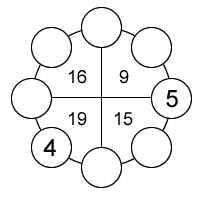Select PagePrintable version

The number within the four sectors of the outer circle is equal to the sum of the three numbers in its sector.  The numbers in the individual circles can only be 0 to 8 and each number can be used only once. The total sum for all the numbers in the outer circle is equal to 36.  Two numbers have been provided to get you started.  Find the remaining six numbers.### Solution### Solution explanationComments on solving the puzzle; a process of eliminating possible values as answers:

• C & F are given as 5 & 4 respectively and their values can not reused.
• Starting at Equation (1), unknowns A & B can be equal to 1 or 3 only as shown. Therefore, D, E, G & H can not be equal to 1, 3, 4 or 5.
• Equation (2): unknowns D & E can be equal to 2 or 8 only as shown. All other combinations include either a 1, 3, 4 or 5 value.
• Equation (3): unknowns E & G can be equal to 6, 7, 8 or 9 as shown. Therefore, comparing with Equation (2), E can only be equal to 8 & D is equal to 2 & G is equal to 7.
• Equation (4): unknowns G, H & A can be equal to 3, 6 or 7 as shown. Comparing Equations (1), (2) & (3) and G is equal to 7. Then H must be equal to 6, A equal to 3 & B equal to 1. The value 9 is not used in the final solution of this puzzle example.

Now insert your final answers into the original puzzle and verify the resulting sums.

## Feedback

There are more than one way of doing these puzzles and may well be more than one answer.  Please let me and others know what alternatives you find by commenting below.  We also welcome general comments on the subject and any feedback you’d like to give.   If you have a question that need a response from me or you would like to contact me privately, please use the contact form.

## Get more puzzles!

If you’ve enjoyed doing the puzzles, consider ordering the book; 150+ of the best puzzles in a handy pocket sized format. Click here for full details.